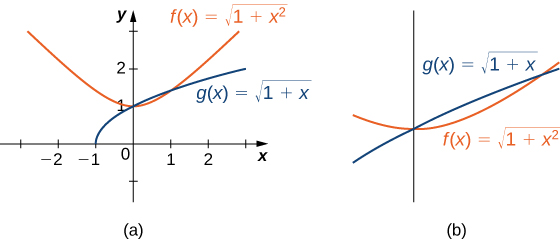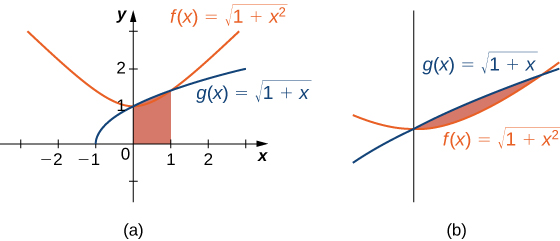5.2 The definite integral  (Page 5/16)

 Page 5 / 16

Using the properties of the definite integral

Use the properties of the definite integral to express the definite integral of $f\left(x\right)=-3{x}^{3}+2x+2$ over the interval $\left[-2,1\right]$ as the sum of three definite integrals.

Using integral notation, we have ${\int }_{-2}^{1}\left(-3{x}^{3}+2x+2\right)dx.$ We apply properties 3. and 5. to get

$\begin{array}{cc}{\int }_{-2}^{1}\left(-3{x}^{3}+2x+2\right)dx\hfill & ={\int }_{-2}^{1}-3{x}^{3}dx+{\int }_{-2}^{1}2xdx+{\int }_{-2}^{1}2dx\hfill \\ & =-3{\int }_{-2}^{1}{x}^{3}dx+2{\int }_{-2}^{1}xdx+{\int }_{-2}^{1}2dx.\hfill \end{array}$

Use the properties of the definite integral to express the definite integral of $f\left(x\right)=6{x}^{3}-4{x}^{2}+2x-3$ over the interval $\left[1,3\right]$ as the sum of four definite integrals.

$6{\int }_{1}^{3}{x}^{3}dx-4{\int }_{1}^{3}{x}^{2}dx+2{\int }_{1}^{3}xdx-{\int }_{1}^{3}3dx$

Using the properties of the definite integral

If it is known that ${\int }_{0}^{8}f\left(x\right)dx=10$ and ${\int }_{0}^{5}f\left(x\right)dx=5,$ find the value of ${\int }_{5}^{8}f\left(x\right)dx.$

By property 6.,

${\int }_{a}^{b}f\left(x\right)dx={\int }_{a}^{c}f\left(x\right)dx+{\int }_{c}^{b}f\left(x\right)dx.$

Thus,

$\begin{array}{ccc}{\int }_{0}^{8}f\left(x\right)dx\hfill & =\hfill & {\int }_{0}^{5}f\left(x\right)dx+{\int }_{5}^{8}f\left(x\right)dx\hfill \\ \hfill 10& =\hfill & 5+{\int }_{5}^{8}f\left(x\right)dx\hfill \\ \hfill 5& =\hfill & {\int }_{5}^{8}f\left(x\right)dx.\hfill \end{array}$

If it is known that ${\int }_{1}^{5}f\left(x\right)dx=-3$ and ${\int }_{2}^{5}f\left(x\right)dx=4,$ find the value of ${\int }_{1}^{2}f\left(x\right)dx.$

−7

Comparison properties of integrals

A picture can sometimes tell us more about a function than the results of computations. Comparing functions by their graphs as well as by their algebraic expressions can often give new insight into the process of integration. Intuitively, we might say that if a function $f\left(x\right)$ is above another function $g\left(x\right),$ then the area between $f\left(x\right)$ and the x -axis is greater than the area between $g\left(x\right)$ and the x -axis. This is true depending on the interval over which the comparison is made. The properties of definite integrals are valid whether $a or $a>b.$ The following properties, however, concern only the case $a\le b,$ and are used when we want to compare the sizes of integrals.

Comparison theorem

1. If $f\left(x\right)\ge 0$ for $a\le x\le b,$ then
${\int }_{a}^{b}f\left(x\right)dx\ge 0.$
2. If $f\left(x\right)\ge g\left(x\right)$ for $a\le x\le b,$ then
${\int }_{a}^{b}f\left(x\right)dx\ge {\int }_{a}^{b}g\left(x\right)dx.$
3. If m and M are constants such that $m\le f\left(x\right)\le M$ for $a\le x\le b,$ then
$\begin{array}{cc}\\ \\ m\left(b-a\right)\hfill & \le {\int }_{a}^{b}f\left(x\right)dx\hfill \\ & \le M\left(b-a\right).\hfill \end{array}$

Comparing two functions over a given interval

Compare $f\left(x\right)=\sqrt{1+{x}^{2}}$ and $g\left(x\right)=\sqrt{1+x}$ over the interval $\left[0,1\right].$

Graphing these functions is necessary to understand how they compare over the interval $\left[0,1\right].$ Initially, when graphed on a graphing calculator, $f\left(x\right)$ appears to be above $g\left(x\right)$ everywhere. However, on the interval $\left[0,1\right],$ the graphs appear to be on top of each other. We need to zoom in to see that, on the interval $\left[0,1\right],g\left(x\right)$ is above $f\left(x\right).$ The two functions intersect at $x=0$ and $x=1$ ( [link] ).(a) The function f ( x ) appears above the function g ( x ) except over the interval [ 0 , 1 ] (b) Viewing the same graph with a greater zoom shows this more clearly.

We can see from the graph that over the interval $\left[0,1\right],g\left(x\right)\ge f\left(x\right).$ Comparing the integrals over the specified interval $\left[0,1\right],$ we also see that ${\int }_{0}^{1}g\left(x\right)dx\ge {\int }_{0}^{1}f\left(x\right)dx$ ( [link] ). The thin, red-shaded area shows just how much difference there is between these two integrals over the interval $\left[0,1\right].$(a) The graph shows that over the interval [ 0 , 1 ] , g ( x ) ≥ f ( x ) , where equality holds only at the endpoints of the interval. (b) Viewing the same graph with a greater zoom shows this more clearly.

Average value of a function

We often need to find the average of a set of numbers, such as an average test grade. Suppose you received the following test scores in your algebra class: 89, 90, 56, 78, 100, and 69. Your semester grade is your average of test scores and you want to know what grade to expect. We can find the average by adding all the scores and dividing by the number of scores. In this case, there are six test scores. Thus,

find the domain and range of f(x)= 4x-7/x²-6x+8
find the range of f(x)=(x+1)(x+4)
-1, -4
Marcia
That's domain. The range is [-9/4,+infinity)
Jacob
If you're using calculus to find the range, you have to find the extrema through the first derivative test and then substitute the x-value for the extrema back into the original equation.
Jacob
Good morning,,, how are you
d/dx{1/y - lny + X^3.Y^5}
How to identify domain and range
hello
Akpevwe
He,,
Harrieta
hi
Dr
hello
velocity
I only talk to girls
Dr
women are smart then guys
Dr
Smarter
sorry
Dr
Dr
:(
Shun
was up
Dr
hello
is it chatting app?.. I do not see any calculus here. lol
Find the arc length of the graph of f(x) = In (sinx) on the interval [Π/4, Π/2].
Sand falling freely from a lorry form a conical shape whose height is always equal to one-third the radius of the base. a. How fast is the volume increasing when the radius of the base is (1m) and increasing at the rate of 1/4cm/sec Pls help me solve
show that lim f(x) + lim g(x)=m+l
list the basic elementary differentials
Differentiation and integration
yes
Damien
proper definition of derivative
the maximum rate of change of one variable with respect to another variable
terms of an AP is 1/v and the vth term is 1/u show that the sum of uv terms is 1/2(uv+1)
what is calculus?
calculus is math that studies the change in math, such as the rate and distance,
Tamarcus
what are the topics in calculus
Augustine
what is limit of a function?
what is x and how x=9.1 take?
what is f(x)
the function at x
Marc
also known as the y value so I could say y=2x or f(x)= 2x same thing just using functional notation your next question is what is dependent and independent variables. I am Dyslexic but know math and which is which confuses me. but one can vary the x value while y depends on which x you use. also
Marc
up domain and range
Marc
enjoy your work and good luck
Marc
I actually wanted to ask another questions on sets if u dont mind please?
Inembo
I have so many questions on set and I really love dis app I never believed u would reply
Inembo
Hmm go ahead and ask you got me curious too much conversation here
am sorry for disturbing I really want to know math that's why *I want to know the meaning of those symbols in sets* e.g n,U,A', etc pls I want to know it and how to solve its problems
Inembo
and how can i solve a question like dis *in a group of 40 students, 32 offer maths and 24 offer physics and 4 offer neither maths nor physics , how many offer both maths and physics*
Inembo
next questions what do dy mean by (A' n B^c)^c'
Inembo
The sets help you to define the function. The function is like a magic box where you put inside stuff(numbers or sets) and you get out the stuff but in different shapes (forms).
I dont understand what you wanna say by (A' n B^c)^c'
(A' n B (rise to the power of c)) all rise to the power of c
Inembo
Aaaahh
Ok so the set is formed by vectors and not numbers
A vector of length n
But you can make a set out of matrixes as well
I I don't even understand sets I wat to know d meaning of all d symbolsnon sets
Inembo
High-school?
yes
Inembo
am having big problem understanding sets more than other math topics
Inembo
So f:R->R means that the function takes real numbers and provides real numer. For ex. If f(x) =2x this means if you give to your function a real number like 2,it gives you also a real number 2times2=4
pls answer this question *in a group of 40 students, 32 offer maths and 24 offer physics and 4 offer neither maths nor physics , how many offer both maths and physics*
Inembo
If you have f:R^n->R^n you give to your function a vector of length n like (a1,a2,...an) where all a1,.. an are reals and gives you also a vector of length n... I don't know if i answering your question. Otherwise on YouTube you havr many videos where they explain it in a simple way
I would say 24
Offer both
Sorry 20
Actually you have 40 - 4 =36 who offer maths or physics or both.
I know its 20 but how to prove it
Inembo
You have 32+24=56who offer courses
56-36=20 who give both courses... I would say that
solution: In a question involving sets and Venn diagram, the sum of the members of set A + set B - the joint members of both set A and B + the members that are not in sets A or B = the total members of the set. In symbolic form n(A U B) = n(A) + n (B) - n (A and B) + n (A U B)'.
Mckenzie
In the case of sets A and B use the letters m and p to represent the sets and we have: n (M U P) = 40; n (M) = 24; n (P) = 32; n (M and P) = unknown; n (M U P)' = 4
Mckenzie
Now substitute the numerical values for the symbolic representation 40 = 24 + 32 - n(M and P) + 4 Now solve for the unknown using algebra: 40 = 24 + 32+ 4 - n(M and P) 40 = 60 - n(M and P) Add n(M and P), as well, subtract 40 from both sides of the equation to find the answer.
Mckenzie
40 - 40 + n(M and P) = 60 - 40 - n(M and P) + n(M and P) Solution: n(M and P) = 20
Mckenzie
thanks
Inembo
Simpler form: Add the sums of set M, set P and the complement of the union of sets M and P then subtract the number of students from the total.
Mckenzie
n(M and P) = (32 + 24 + 4) - 40 = 60 - 40 = 20
MckenzieBy Edward Biton